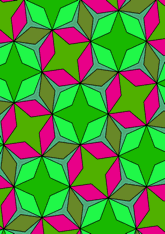# Derived from 3₂.4.3.4, with digons added and two starsdata159/D3434C1

## Geometry

• The symmetry group of the tiling is 442 (p4).
• All the internal angles of the constituent polygons are a multiple of 15°.
• Contains three regular two-pointed star polygons with vertex angle of 45°.
• Contains one regular three-pointed star polygon with vertex angle of 15°.
• Contains two regular four-pointed star polygons with vertex angle of 45°.
• The tiling satisfies the two-colour condition.
• The tiling is edge-to-edge.
• As drawn, contains about 179 polygons.

## References

Publications referenced:
1. Uniform Star Polygons with Digons of B A Wichmann. The World of Patterns, World Scientific, 2001. ISBN 981024619. [wich2] {Only new patterns ascribed to this.}

v53